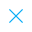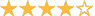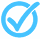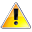Call Support +91-85588-96644

Keep me logged in
You can't leave Captcha Code empty
By submitting this form, you agree to the Terms & Privacy Policy.
ORTests given

OR# Plotting Straight Line Graphs| By TCY

Questions

Time

Highest
score

Level

## ENGLISH

Language

Topics Covered:y = mx + cCoordinate GeometryCoordinatesSolving GraphicallyGraph of Equation ax + by + c = 0Various Forms of the Equation a LineTheory of EquationsLinear EquationsLinear Equations in One and Two VariablesPlotting Solutions of Linear EquationsThis is a concept-building practice test and may not have exact structure as you would expect in the actual exam. Please exercise your discretion to attempt it or go to structured Featured Section.

Description:
Plotting a Straightline Graph
•